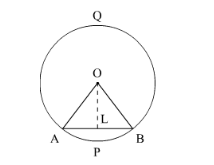# AB is a chord of a circle with centre O and radius 4 cm.Question:

AB is a chord of a circle with centre O and radius 4 cm. AB is of length 4 cm. Find the area of the sector of the circle formed by chord AB.

Solution:

We have to find the area of the sector AOB formed by the chord AB.We have $O A=4 \mathrm{~cm}$ and $A B=4 \mathrm{~cm}$. So,

$A L=\frac{A B}{2} \mathrm{~cm}$

$=\frac{4}{2} \mathrm{~cm}$

$=2 \mathrm{~cm}$

Let $\angle A O B=2 \theta$. Then,

$\angle A O L=\angle B O L$

$=\theta$

In $\triangle O L A$, we have

$\sin \theta=\frac{A L}{O A}$

=\frac{2}{4}

$=\frac{1}{2}$

$\theta=\sin ^{-1} \frac{1}{2}$

$=30^{\circ}$

Hence, $\angle A O B=60^{\circ}$

Now, using the value of $\angle A O B$ and $r$ we will find the area of sector $A O B$,

$A=\frac{\theta}{360^{\circ}} \times \pi r^{2}$

$=\frac{60^{\circ}}{360^{\circ}} \times \pi \times 4 \times 4 \mathrm{~cm}^{2}$

$=\frac{8 \pi}{3} \mathrm{~cm}^{2}$